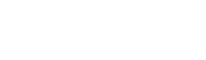# 【OpenCV】the similarity of images using the AKAZE detector

スポンサーリンク

Hello, this is Yuki (@engineerblog_Yu), a student engineer.

The image above shows 100 feature points plotted by OpenCV.

This time, we verified whether the feature points can be matched even if the image is rotated or scaled down.

The following is the coding part.

スポンサーリンク

## Coding

import cv2

img2 = cv2.resize(img2,(300,280))

height = img2.shape

width = img2.shape

center = (int(width/2), int(height/2))

angle = 45.0

scale = 1.0

trans = cv2.getRotationMatrix2D(center, angle , scale)

img2 = cv2.warpAffine(img2, trans, (width,height))

akaze = cv2.AKAZE_create()

kp1,des1 = akaze.detectAndCompute(img1,None)
kp2,des2 = akaze.detectAndCompute(img2,None)

bf = cv2.BFMatcher(cv2.NORM_HAMMING, crossCheck=True)

matches = bf.match(des1,des2)

matches = sorted(matches, key = lambda x:x.distance)

img3 = cv2.drawMatches(img1, kp1, img2, kp2, matches[:30], None, flags = cv2.DrawMatchesFlags_NOT_DRAW_SINGLE_POINTS)
cv2_imshow(img3)

In this case, we sorted the characteristic points in order of importance and extracted 30 of them.

You can see that the feature points roughly match those of the original image rotated and scaled down.

## Display of coordinates of similar feature points

img1_pt = [list(map(int, kp1[m.queryIdx].pt)) for m in matches]

img2_pt = [list(map(int, kp2[m.trainIdx].pt)) for m in matches]

print(img1_pt)

print(img2_pt)

output:

Thank you for reading to the end!

スポンサーリンク
Yukiをフォローするautotech
タイトルとURLをコピーしました